# Hawkes Processes: First Derivatives

A Hawkes process is a representation of a measure of new events, λ. Which can have the following interpretation; λ = 𝔼[number of events from t to t + 𝛿]. The Hawkes process is a heterogeneous poisson process; because λ changes. The original publication gave a method to find parameters that involved a spectral decomposition. The first order of a functional with respect to the parameters.

The functional takes the form;Single label trigger kernel K with a non-mobile base measure μ.

To complete the expression;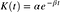Exponential decay, which makes performing the sum feasible.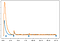An online multi-label Hawkes Process which goes to timestep 18.

# Use cases

A probability model makes more sense when dealing with missing data instead of a prediction model. Publications have deployed a Hawkes process to find motifs in DNA sequences, high frequency trading, spread of infectious disease, rate of active shooter situations, or the information exchanges on social networks. The parameters are interpretable. Kernels are modular; as the observation’s entropy increases different influence patterns can be learned.

# Derivatives

The log probability of the exemplars given Θ ={α, β, μ}; is provided. Where α is the strength of an item, while β is the distance it influences.The fully expanded log likelihood given the kernel.

Typical notation requires upper case letters signify matrices, but here T is the total time while N as the number of events. These should be functions. Taking the derivative of the expression with respect to each of the parameters.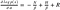The formulas have English translation that corresponds to what the calculation is performing. In other words, the derivatives are interpretable.

Here are summary values which make the computation quicker.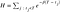The summary values might be invalid if improper data types hold the values.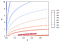An example of the topology of a loss surface

Multiple libraries to implementing point processes exist. Most are specific to a field or application; spike trains in neurology, responding to text conversations, or imputing missing data. It ties back to modeling interactions in social sciences. Here is the toolkit implementing the online version. An alternative version with more complex optimization techniques exists, but the toolkit provided can be easier to start with.

awake, software engineer.